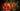# JavaScript 中的值分类

## 前置知识

### 基础类型与对象(object)

JavaScript 中的所有值都是基础类型或对象两者中的一个。

• undefined
• null
• Booleans
• Numbers
• Strings

``````var str = 'abc';
str.foo = 123; // 试着添加属性 "foo"
// -> 123
str.foo; // 没有改变
// -> undefined``````

``'abc' === 'abc'; // true``

``````var obj = {};
obj.foo = 123; // 试着添加属性 "foo"
// -> 123
obj.foo; // 属性 "foo" 被添加
// -> 123``````

``````{} === {}; // false

var obj = {};
obj === obj; // true``````

``````typeof new String('abc'); // 'object'
typeof 'abc'; // 'string'
new String('abc') === 'abc'; // false``````

``String.prototype.indexOf === ''.indexOf; // true``

### 术语：原型与原型对象

1. 一方面，对象之间存在原型联系。每个对象都有隐含属性[[Prototype]]指向它的 prototype(原型) 或者 `null`。原型是对象的延续。如果引用对象的属性却没有找到，将继续搜索前者(即在原型上查找)。不同的对象可以有相同的原型。
2. 另一方面，如果一个类型是构造方法 `Foo` 的实例，那么该构造方法拥有原型对象 `Foo.prototype` 的属性。

• 方法 `Object.getPrototypeOf(obj)` 返回对象 `obj` 的原型：
``Object.getPrototypeOf({}) === Object.prototype; // true``
• 方法 `Object.create(proto)` 创建一个原型为 `proto` 的空对象。
``Object.create(Object.prototype); // {}``
• 方法 `proto.isPrototypeOf(obj)` 是用来确定对象 `proto` 是否是对象 `obj` 的原型。如果 `proto``obj` 的原型(或原型的原型)，方法 `proto.isPrototypeOf(obj)` 返回 `true`
``Object.prototype.isPrototypeOf({}); // true``

### 属性“constructor”(构造方法)

``````function Foo() {}
Foo.prototype.constructor === Foo; // true
RegExp.prototype.constructor === RegExp; // true``````

``````new Foo().constructor; // [Function: Foo]

/abc/.constructor; // [Function: RegExp]``````

## 值分类

• [[Class]] 是一个内部属性，表示将对象分类的一个字符串；
• `typeof` 是一个对基础类型分类的操作符，并帮助我们区别对象；
• `instanceof` 是对对象进行分类的操作符；
• `Array.isArray()` 是用来判断值是否是数组的函数。

### [[Class]] 属性

[[Class]] 是一个值为下面字符串的内部属性：

"Arguments", "Array", "Boolean", "Date", "Error", "Function", "JSON", "Math", "Number", "Object", "RegExp", "String"

• "[object Undefined]" 如果 `this``undefined`,
• "[object Null]" 如果 `this``null`,
• "[object " + obj.[[Class]] + "]" 如果 `this` 是一个对象 `obj`.
• 基础类型被转换为对象，就像之前的规则一样处理。

``````Object.prototype.toString.call(undefined);
// -> '[object Undefined]'
Object.prototype.toString.call(Math);
// -> '[object Math]'
Object.prototype.toString.call({});
// -> '[object Object]'``````

``````function getClass(x) {
const str = Object.prototype.toString.call(x);
return /^\[object (.*)\]\$/.exec(str);
}``````

``````getClass(null); // 'Null'

getClass({}); // 'Object'

getClass([]); // 'Array'

getClass(JSON); // 'JSON'

(function () {
return getClass(arguments);
})(); // 'Arguments'

function Foo() {}
getClass(new Foo()); // 'Object'``````

### typeof 操作符

``typeof value;``

`typeof` 操作 `null` 返回 `"object"` 是一个 bug。这无法被修复，因为修复意味着破话现有代码。注意，函数也是对象，但是 `typeof` 区分了它们。另一方面，数组却被它视为对象。

### instanceof 操作符

`instanceof` 检查值是否是一个类型的实例：

``value instanceof Type;``

``````function myInstanceof(value, Type) {
return Type.prototype.isPrototypeOf(value);
}``````

``````'' instanceof String; // false
'' instanceof Object; // false``````

### Array.isArray()

`Array.isArray()` 的出现是因为浏览器的一个特殊问题：每一个 `frame` 都有自己的全局环境。举一个例子：给定 `frame` A 和 `frame` B(它们在同一个页面)。`frame` A 可以添加一个值到 `frame` B 的代码中。然后 B 的代码不能使用 `instanceof Array` 来检查这个值是否是一个数组，因为它是 B 的数组不同于 A 的数组。例子：

``````<html>
<script>
// test() is called from the iframe
function test(arr) {
const iframeWin = frames;
console.log(arr instanceof Array); // false
console.log(arr instanceof iframeWin.Array); // true
console.log(Array.isArray(arr)); // true
}
</script>
<body>
<iframe></iframe>
<script>
// Fill the iframe
const iframeWin = frames;
iframeWin.document.write('<script>window.parent.test([])</' + 'script>');
</script>
</body>
</html>``````

## 内置的原型对象

### Object.prototype

`Object.prototype` 就像一个空对象：他没有任何可枚举的自有属性(它的方法都是不可枚举的)。

``````Object.prototype; // {}
Object.keys(Object.prototype); // []``````

``````getClass(Object.prototype); // 'Object'
typeof Object.prototype; // 'object'``````

``Object.prototype instanceof Object; // false``

``Object.getPrototypeOf(Object.prototype); // null``

``````getClass({}); // 'Object'
typeof {}; // object'
{} instanceof Object; // true``````

### Function.prototype

`Function.prototype` 本身就是一个函数。它接受任何参数并返回 `undefined`

``Function.prototype('a', 'b', 1, 2); // undefined``

``typeof Function.prototype； // 'function'``

[[Class]] 属性也说明相同的事：

``getClass(Function.prototype); // 'Function'``

``Function.prototype instanceof Function; // false``

``````Object.getPrototypeOf(Function.prototype) === Object.prototype;
// -> true``````

``````typeof function () {}; // 'function'
getClass(function () {}); // 'Function'
function () {} instanceof Function; // true``````

`Function` 也是一个函数：

``````typeof Function; // 'function'
getClass(Function); // 'Function'
Function instanceof Function; // true``````

### Array.prototype

`Array.prototype` 本身是一个空数组：它以这种方法显示且长度为 0。

``````Array.prototype; // []
Array.prototype.length; // 0``````

[[Class]] 也证明它是数组：

``getClass(Array.prototype); // 'Array'``

``Array.isArray(Array.prototype); // true``

``Array.prototype instanceof Array; // false``

### RegExp.prototype

`RegExp.prototype` 是一个匹配所有内容的正则表达式：

``````RegExp.prototype.test('abc'); // true
RegExp.prototype.test(''); // true``````

`String.prototype.match` 也接受 `RegExp.prototype`，它通过 [[Class]] 检查其参数是否是正则表达式。对于正则表达式和原型对象，该检查是令人信服的：

``````getClass(/abc/); // 'RegExp'
getClass(RegExp.prototype); // 'RegExp'``````

`RegExp.prototype` 等价于“空正则表达式”。这种表达式有两种创建方式：

``````new RegExp(''); // 通过构造方法方式
/(?:)/; // 字面量方式``````

``````new RegExp('').exec('abc');
// -> [ '', index: 0, input: 'abc' ]
/(?:)/.exec('abc');
// -> [ '', index: 0, input: 'abc' ]``````

``````/()/.exec("abc")
// ->
[ '',  // index 0
'',  // index 1
index: 0,
input: 'abc' ]``````

``````new RegExp(''); // /(?:)/
RegExp.prototype; // /(?:)/``````

### Date.prototype

`Date.prototype` 也是一个日期类型：

``````getClass(new Date()); // 'Date'
getClass(Date.prototype); // 'Date'``````

• Date 对象包含一个数字，表示一个毫秒内的特定时刻。这样的数字称为时间值。时间值也可以是 `NaN`，表示 Date 对象不表示特定的时刻。
• ECMAScript 规定时间是自 UTC 时间 1970 年 1 月 1 日 00:00:00 起，以毫秒为单位。

``````const d = new Date(); // now

d.valueOf(); /// 1347035199049
Number(d); // 1347035199049``````

``````const now = new Date();
now.getTime(); // 1560500640506

Date.now(); // 1560500676490``````

`Date.prototype` 的时间值是 `NaN`

``````Date.prototype.valueOf(); // NaN
Number(Date.prototype); // NaN``````

`Date.prototype` 显示的是无效的时间，可以通过 `NaN` 创建相同的时间：

``````Date.prototype; // Invalid Date
new Date(NaN); // Invalid Date``````

### Number.prototype

`Number.prototype``new Number(0)` 大约相同：

``Number.prototype.valueOf(); // 0``

``+Number.prototype; // 0``

``+new Number(0); // 0``

### String.prototype

`String.prototype``new String('')` 大约相同：

``String.prototype.valueOf(); // ''``

``'' + String.prototype; // ''``

``'' + new String(0); // ''``

### Boolean.prototype

`Boolean.prototype``new Boolean(false)`大约相同：

``Boolean.prototype.valueOf(); // false``

``````!!Boolean.prototype; //true
!!new Boolean(false); // true
!!new Boolean(true); // true``````